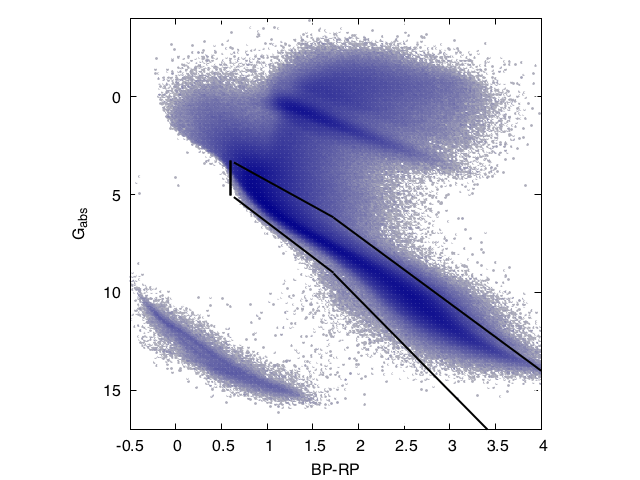# 10.13.2 Properties of the input data

The processed input time series are the $G$, $G_{\rm BP}$, and $G_{\rm RP}$ time series obtained with the operators chain described in Section 10.2.3 and corresponding to the ExtremeErrorCleaningMagnitudeDependent tag. The different steps of the code require that for each transit, the $G$, $G_{\rm BP}$, and $G_{\rm RP}$ photometric measurements are simultaneously available. For this reason, we applied to the three time series a further filter that removes all transits in which one or two of the three photometric measurements are missing. The input sources were selected by adopting the following criteria:

1. 1.

the $G$, $G_{\rm BP}$, and $G_{\rm RP}$ time series have a sampling that allows the extraction of sub-series satisfying the condition in Equation 10.17;

2. 2.

the relative parallax error is less than 0.2;

3. 3.

the location of the star in the colour-absolute magnitude diagram is close to the late-type main sequence as identified by the following conditions:

 $\left\{\begin{array}[]{l}0.6\leq(G_{\mathrm{BP}}-G_{\mathrm{RP}})\leq 1.7\\ \mbox{M_{\rm G}}\leq 2.873+3.545\,(G_{\mathrm{BP}}-G_{\mathrm{RP}})\\ \mbox{M_{\rm G}}\geq 1.7225+2.572\,(G_{\mathrm{BP}}-G_{\mathrm{RP}})\end{% array}\right.$ (10.14)

or

 $\left\{\begin{array}[]{l}G_{\mathrm{BP}}-G_{\mathrm{RP}}>1.7\\ \mbox{M_{\rm G}}\leq 0.853+4.733\,(G_{\mathrm{BP}}-G_{\mathrm{RP}})\\ \mbox{M_{\rm G}}\geq 0.235+3.441\,(G_{\mathrm{BP}}-G_{\mathrm{RP}}).\end{% array}\right.$ (10.15)

The first criterion ensures that each star has a sufficient number of photometric measurements to perform a period search in different segments of the time series, which is an effective strategy to take the AR evolution into account. The second and third criteria ensure that the analysed sample includes main-sequence stars and T Tauri stars of class II and III, as shown in Figure 10.29.Figure 10.29: Observational Hertzsprung–Russell diagram of the input sources, with the borders of the area used to select the solar-like variable candidates marked by a solid line.